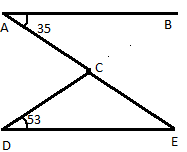QUESTION

# Find the angle $\angle DCE$?Hint: In order to find the required angle first we have to determine $\angle DEC$ in $\Delta DCE,$ we will determine it by using the alternate angle theorem which states that alternate angles are angles that are in opposite positions relative to a transversal intersecting two lines.

We have to find $\angle DCE$
First, we have to find $\angle DEC$
So, we know that the alternate angles are angles that are in opposite positions relative to a transversal intersecting two lines.
Here alternate angles are $\angle BAE{\text{ and }}\angle AED$
According to the alternate angle theorem
$\angle BAE = \angle AED = {35^0}..............\left( 1 \right)$
Now, consider $\Delta CDE,$ where $\angle CDE = {53^0}$ and $\angle CED = {35^0}{\text{ }}\left[ {{\text{from equation }}\left( 1 \right)} \right]$
As we know that in triangle sum of angles must be ${180^0}$
$\therefore {\text{ In }}\Delta CDE \\ \angle CDE + \angle CED + \angle DCE = {180^0} \\$
Substitute the values of $\angle CDE,\angle CED,$ we have
$\Rightarrow {53^0} + {35^0} + \angle DCE = {180^0} \\ \Rightarrow {88^0} + \angle DCE = {180^0} \\ \Rightarrow \angle DCE = {180^0} - {88^0} \\ \Rightarrow \angle DCE = {92^0} \\$
Hence, the required angle $\angle DCE{\text{ is }}{92^0}.$

Note: In order to solve these types of questions, you need to learn all the properties of angles and parallel lines. Some of these properties are alternate angle property, corresponding angle property, vertically opposite angles etc. Also remember that the sum of interior angles of a triangle is 180 degrees while that of the sum of interior angles of squares is 360 degrees.